﻿ Heating due to the Iron Loss(Temperature-dependent materials)Examples | Product | Murata Software Co., Ltd.# Example4Heating due to the Iron Loss(Temperature-dependent materials)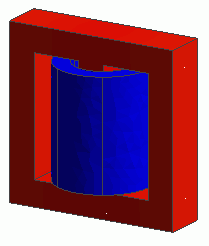General

• A coil is wound on a core. The heating due to the iron loss is analyzed.

• The temperature dependencies of the core’s permeability and conductivity are taken into account.
This is bi-directional between magnetic field and thermal analyses and will go back and forth until the calculation reaches the convergence.
The calculation time is much longer than that of no temperature dependency.

• The vectors of the magnetic field, the loss in the core, and the temperature distribution by the heating are solved.

• Unless specified in the list below, the default conditions will be applied.

### Analysis Space

 Item Settings Analysis Space 3D Model unit mm

### Analysis Conditions

 Item Settings Solvers Magnetic Field Analysis [Gauss] Thermal Analysis [Watt] Analysis Type Magnetic field analysis: Harmonic analysis Thermal analysis: Steady-state analysis Options N/A

The frequency of the current is set to 50[kHz].

 Tab Setting Item Settings Mesh Tab Frequency-Dependent Meshing Reference frequency: 50×10^3[Hz] Surface Treatment for Conductors: Generate skin meshes Harmonic analysis Frequency Sweep Type: Single frequency Frequency: 50×10^3[Hz]

### Model

A coil is wound on a core.

The bodies of a core (Core) and a loop coil (Coil) are defined.

The automatically created ambient air is analyzed only in the magnetic field analysis, not in the thermal analysis.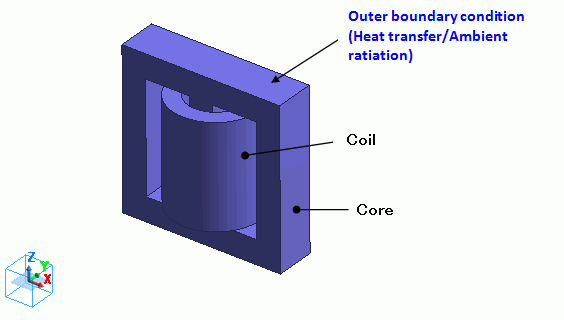### Body Attributes and Materials

 Body Number/Type Body Attribute Name Material Name 5/Solid Coil2 008_Cu * 7/Solid Coil1 008_Cu * 6/Solid Core Core

* Available from the Material DB

Body attribute is set up as follows to apply current to the loop coil.

 Body Attribute Name Tab Settings Coil Current Waveform: AC Current: 0.1[A] Turns: 100[Turns] Induced current: No setting Direction: Loop Coil/Magnetic Field Direction Direction Vector of Magnetic Field: X=0, Y=0, Z=1

The material properties of the core are set up as follows.

Permeability and conductivity are temperature-dependent.

The loss is defined in the iron loss table.

Material Name

Tab

Settings

Core

Permeability

Temperature Dependency: Yes

[Temperature-Relative Permeability] Table

 Temperature [deg] Relative Permeability 0 3000 100 2700 500 1500 1000 30

Electric Conductivity

Conductivity Type: Conductor

Temperature Dependency: Yes

[Temperature-Conductivity] Table

 Temperature [deg] Electric Conductivity 0 0.1 100 0.06 500 0.02 1000 0.01

Thermal Conductivity

Thermal Conductivity: 10[W/m/deg]

Iron loss

Iron Loss Calculation Type: Iron loss table

Frequency: 5×10^4[Hz]

[Magnetic flux density-Loss density] Table *

 Magnetic Flux Density [T] Joule Loss Density [W/m^3] 50×10-3 5×10^3 500×10-3 500×10^3

* This is not the actual material’s property.

Press the Graph button. The following graph will show up.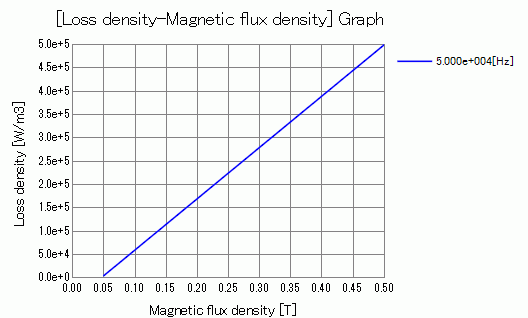### Boundary Conditions

“Natural convection (automatic calculation)” is set on the outer boundary condition (surrounding the coil and core in the case of the thermal analysis).

 Boundary Condition Name/Topology Tab Boundary Condition Type Settings Outer Boundary Condition * Thermal Heat Transfer/Ambient Radiation Select “Natural convection (automatic calculation)”. Room Temperature : 25[deg]

To set Outer Boundary Condition, go to the [Model] tab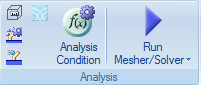and click [Outer Boundary Condition]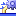.

* The correction coefficient for the natural convection is calculated automatically. See [Heat Transfer/Ambient Radiation] for more information.

### Results

To see the iron loss, go to the [Results] tabclick [Table]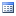.

The joule loss is shown below.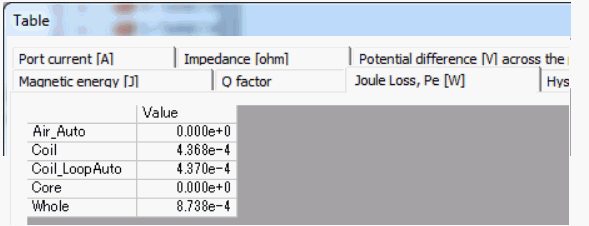The iron loss is shown below.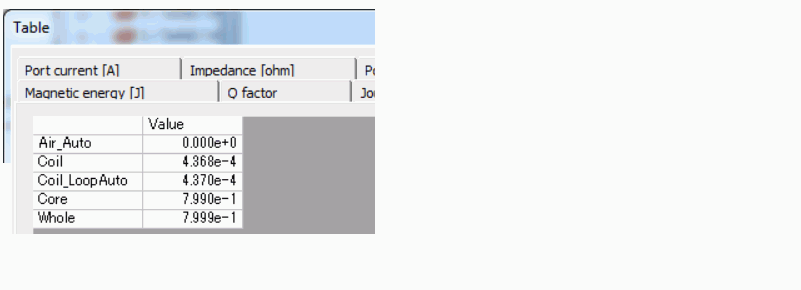The core’s iron loss is approximately 0.8[W].

The joule loss and the hysteresis loss of the core are not given because the the material property is defined on the iron loss table.

The loss characteristics are defined in the iron loss table. Only iron loss is obtained.

If it is defined by the iron loss experimental formula, the joule loss and the hysteresis loss will be output as well.

See [Loss Calculation in the Magnetic Field Analysis] for details of the loss.

The vectors of the magnetic flux density are shown below.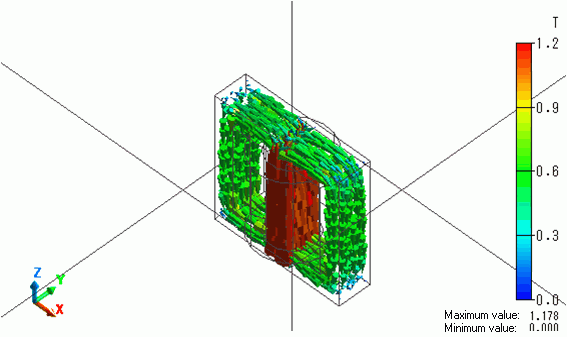The flux density is looping through the core.

The iron loss density is shown below.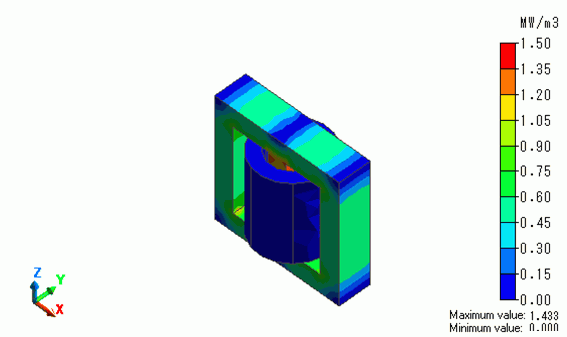The temperature contour as a result of Watt is shown below.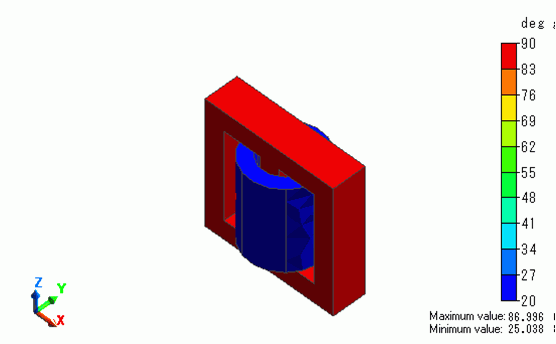The core temperature goes up to around 87[deg].

﻿